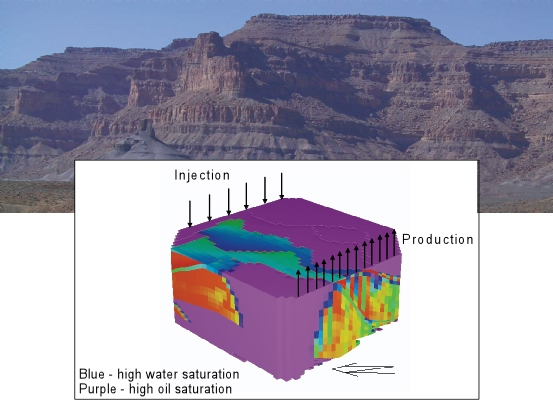# Computational methods for oil recovery

### What is EOR?

In the first stage of oil reservoir production (primary recovery), the oil is extracted by natural drive mechanism, solution gas, water influx, gas cap drive or gas drainage.  In order to maintain reservoir pressure, techniques such as gas or water injection are applied; this is known as secondary recovery.

Tertiary or Enhanced Oil Recovery (EOR) is a generic term that embraces several techniques used to increase the amount of crude oil that can be extracted from an oil field.  These techniques are based on the injection of materials not normally present in the reservoir, and is the most advanced stage of the exploitation of a reservoir.

Primary recovery techniques produce 10–15% of the reservoir’s oil content.  Combining the processes of secondary and tertiary recovery techniques, it is possible to produce 30–60% of the reservoir’s total oil content.

### Mathematical and computational modeling of EOR

Mathematical and computational models permit studying complex systems and natural phenomena that otherwise would be very costly, dangerous or even impossible to study by direct experimentation.  EOR simulations take advantage of mathematical and computational models to design optimal experiments, reducing costs and times of oil production.

In a typical numerical simulation, several millions of unknowns need to be calculated each time step.  Because the simulation’s objective is to reproduce the daily history of an exploited field during, for example, 20 years of operation, we require tens of thousands of time steps to generate the complete production history.  On the other hand, due to the uncertainty of many physical parameters, hundreds of numerical experiments are required.

Parallel computing is an obvious way to accelerate the generation of numerical information and many efforts on this area have been made. Also, with the advent of new and ubiquitous GPU hardware, it is possible to accelerate many of the calculations using modern programming techniques.

### The aim of this course

In this course, a brief introduction to EOR will be presented along with some numerical and computational techniques currently applied in this research field.  The tentative schedule includes:

• Lesson 1:  Introduction to EOR
• Lesson 2: Axiomatic formulation of multiphase systems
• Lesson 3: Numerical methods applied in EOR
• Lesson 4: Computational approaches:  object-oriented and generic programming
• Lesson 5: Some examples; experiences solving two-phase flow models in a GPU